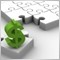Linear regression slope from MT5 to MT4132

Hello,

I have an indicator on MT5 which includes linear regression slope, with the slope function (to get the slope of array x[]) as follows:

double LinRegrSlope(int per)
{
double sum=0.0;
double wsum=0.0;
for(int i=per;i>0;i--)
{
sum+=x[i];
wsum+=x[i]*(per+1-i);
}
double lrs1;
lrs1=6.*(2.*wsum/(per+1)/sum-1.)/(per-1); // normalize to SMA
lrs1 = lrs1*100000.;
if(lrs1>0) lrs1 = MathLog(lrs1);
else {if(lrs1<0)lrs1 = -1.*MathLog(MathAbs(lrs1));
else lrs1 = 0;
}
return(lrs1);
}

This function works normally on my MT5 indicator. However, running it on MT4, the indicator gets "zero divide" on the line: lrs1=6.*(2.*wsum/(per+1)/sum-1.)/(per-1); at the "/sum-1."

Is the function missing something for MT4?

Thank you.8788

anuwat:

Hello,

I have an indicator on MT5 which includes linear regression slope, with the slope function (to get the slope of array x[]) as follows:

This function works normally on my MT5 indicator. However, running it on MT4, the indicator gets "zero divide" on the line: lrs1=6.*(2.*wsum/(per+1)/sum-1.)/(per-1); at the "/sum-1."

Is the function missing something for MT4?

Thank you.

Hi

A quick and dirty solution is to check if (sum-1)  = 0 and make it something like 0.0000000001 instead